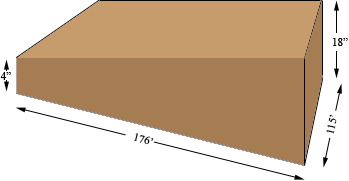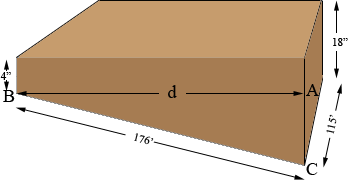SEARCH HOMEMath Central Quandaries & QueriesQuestion from Donnie: I need to know the formula to figure cubic yds of fill dirt to fill an area from 4ft. deep to 18 inches deep this lot is on a slope and I am wanting to level 176 ft long by 4ft. deep the sides is 115 ft. wide the upper side is 18 inches deep.Donnie,The volume is the area of the cross-section times te width of 115 feet. The shape of the cross-section is a trapezoid, a figure bounded by 4 lines, two of which are parallel. The area of a trapezoid is the average of the lengths of the parallel sides times the distance between the parallel sides. You have parallel sides of length 4 and 18 inches but you don't know the distance between them. It should be close to 176' but lets find it more exactly.

Before I begin I am going to change all the dimensions to yards since you asked for the volume in cubic yards. In yards the dimensions arre 176/3 = 58.67, 115/3 = 38.33, 18/36 = 0.50 and 4/36 = 0.11.

To find the distance between the parallel sides d I am going to use Pythagoras theorem.Triangle ABC is a right triangle |BC| = 58.67 yards, |CA| = 0.5 - 0.11 = 0.39 yards and |AB| = d yards. Thus

0.392 + d2 = 58.672

and hence

d = √(58.672 - 0.392) = 58.67 yards.

Thus two 2 decimal places d and |BC| are the same length.

The area of the trapezoidal cross-section is thus

[(0.50 + 0.11)/2] × 58.67 = 17.89 square yards.

Finally the volume of fill you need is 17.89 × 38.33 = 686 cubic yards.

HarleyMath Central is supported by the University of Regina and The Pacific Institute for the Mathematical Sciences.